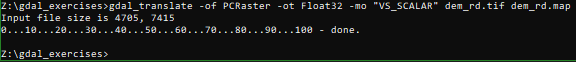## Introduction to GDAL

This tutorial will introduce you to the GDAL command line tools.

### 4. Convert GIS formats

#### 4.1. Convert raster formats

gdal_translate's primary function is to change between image formats. The basic syntax is:

gdal_translate -of FORMAT inputFile outputFile

All supported formats can be found here.

Now we are going to convert the DEM from geoTiff to SAGA format. SAGA is a GIS which has its own -gdal-supportd- binary format with the .sdat extension.

1. Execute the following command:
gdal_translate -of SAGA dem_rd.tif dem_rd.sdat

Some formats need more arguments. PCRaster for examples needs a specification of the data type (boolean, nominal, scalar, etc.). Let's convert the same data to PCRaster format.

2. Execute the following command:
gdal_translate -of PCRaster -ot Float32 -mo "VS_SCALAR" dem_rd.tif dem_rd.mapTo convert to PCRaster format you need to know the data type of the raster. In the example above the DEM is continuous data, so the data type is scalar. If the layer was discrete (classes) then the data type was nominal.

Data Type
-of -mo
Boolean Byte "VS_BOOLEAN"
Nominal Int32 "VS_NOMINAL"
Ordinal Int32 "VS_ORDINAL"
Scalar Float32 or Float64
"VS_SCALAR"
Direction Float32 or Float64
"VS_DIRECTION"
LDD Int32 "VS_LDD"